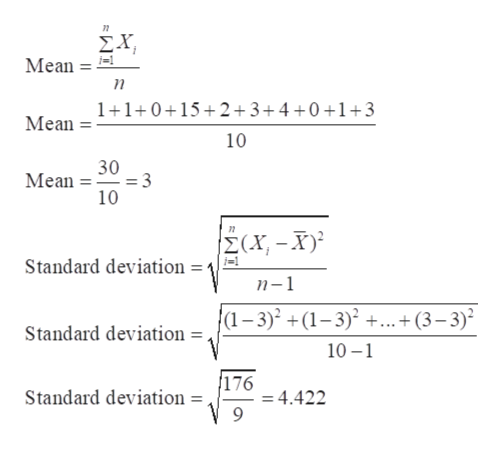# Suppose that a set of ten measurements is drawn randomly. The sample consists of the set {1,1,0,15,2,3,4,0,1,3}. By inspection it is evident that the measurement x=15 appears unusually large in comparison to the rest of the measurements. Calculate the z-score equivalent for the measurement x=15 and determine if it is an outlier.

Question
3 views

Suppose that a set of ten measurements is drawn randomly. The sample consists of the set {1,1,0,15,2,3,4,0,1,3}. By inspection it is evident that the measurement x=15 appears unusually large in comparison to the rest of the measurements. Calculate the z-score equivalent for the measurement x=15 and determine if it is an outlier.

check_circle

Step 1

Given sample
1,1,0,15,2,3,4,0,1,3

To calculate the Z score we need mean and standard deviation using the below mentioned formul...help_outlineImage Transcriptionclose7 Mean Mean- 1+1+0+15+2+3+4+0+1+3 10 30 Mean 3 10 M(X, -X} Standard deviation =' n-1 Standard deviation = /(1-3) +(1-3)2 ...+ (3-3) 10 1 176 = 4.422 9 Standard deviation = fullscreen

### Want to see the full answer?

See Solution

#### Want to see this answer and more?

Solutions are written by subject experts who are available 24/7. Questions are typically answered within 1 hour.*

See Solution
*Response times may vary by subject and question.
Tagged in

### Measures